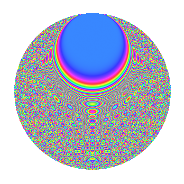# Properties

 Label 1575.2.csLevel 1575 Weight 2 Character orbit cs Rep. character $$\chi_{1575}(46,\cdot)$$ Character field $$\Q(\zeta_{15})$$ Dimension 784 Sturm bound 480

# Related objects

## Defining parameters

 Level: $$N$$ = $$1575 = 3^{2} \cdot 5^{2} \cdot 7$$ Weight: $$k$$ = $$2$$ Character orbit: $$[\chi]$$ = 1575.cs (of order $$15$$ and degree $$8$$) Character conductor: $$\operatorname{cond}(\chi)$$ = $$175$$ Character field: $$\Q(\zeta_{15})$$ Sturm bound: $$480$$

## Dimensions

The following table gives the dimensions of various subspaces of $$M_{2}(1575, [\chi])$$.

Total New Old
Modular forms 1984 816 1168
Cusp forms 1856 784 1072
Eisenstein series 128 32 96

## Trace form

 $$784q + 3q^{2} + 93q^{4} + 5q^{5} - 14q^{7} + 38q^{8} + O(q^{10})$$ $$784q + 3q^{2} + 93q^{4} + 5q^{5} - 14q^{7} + 38q^{8} - 7q^{10} - 12q^{13} - 6q^{14} + 93q^{16} + 3q^{17} - 11q^{19} + 32q^{20} + 12q^{22} + 5q^{23} - 7q^{25} - 44q^{26} - 38q^{28} - 9q^{31} + 40q^{32} + 28q^{34} - 8q^{35} + 11q^{37} + 10q^{38} + q^{40} - 2q^{41} + 36q^{43} + 23q^{44} - 43q^{46} + 5q^{47} - 6q^{49} + 82q^{50} - 70q^{52} + 23q^{53} - 52q^{55} + 60q^{56} - 59q^{58} + 15q^{59} - 13q^{61} + 92q^{62} - 214q^{64} - q^{65} - 45q^{67} + 122q^{68} - 53q^{70} + 10q^{71} + 47q^{73} + 22q^{74} - 228q^{76} - 9q^{77} + q^{79} + 70q^{80} + 28q^{82} + 26q^{83} - 98q^{85} + 41q^{86} - 14q^{88} + 42q^{89} - 38q^{91} + 154q^{92} - 43q^{94} + 57q^{95} - 168q^{97} - 106q^{98} + O(q^{100})$$

## Decomposition of $$S_{2}^{\mathrm{new}}(1575, [\chi])$$ into newform subspaces

The newforms in this space have not yet been added to the LMFDB.

## Decomposition of $$S_{2}^{\mathrm{old}}(1575, [\chi])$$ into lower level spaces

$$S_{2}^{\mathrm{old}}(1575, [\chi]) \cong$$ $$S_{2}^{\mathrm{new}}(175, [\chi])$$$$^{\oplus 3}$$$$\oplus$$$$S_{2}^{\mathrm{new}}(525, [\chi])$$$$^{\oplus 2}$$

## Hecke Characteristic Polynomials

There are no characteristic polynomials of Hecke operators in the database